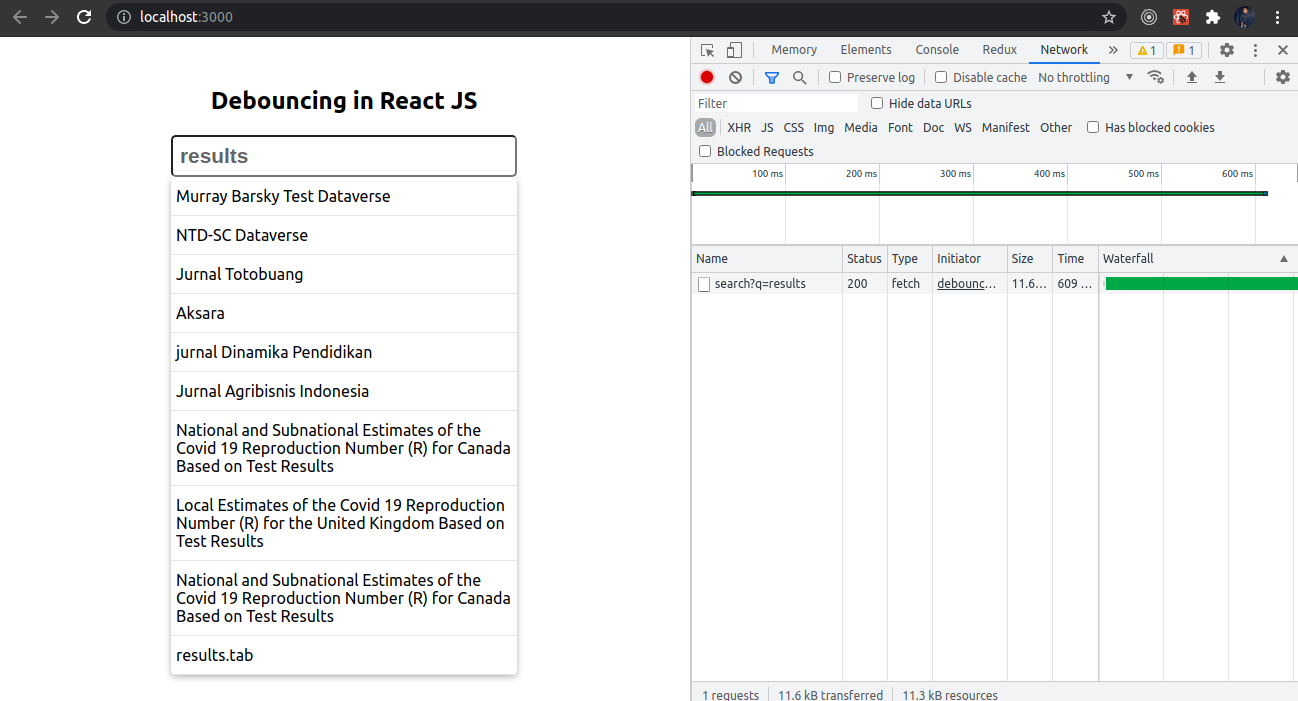# Implement Debouncing in React in 3 Different Ways

Debouncing is used for optimizing the performance of a web app. It is done by limiting the rate of execution of a particular function (also known as rate limiting).

We will learn about debouncing by implementing it on an input box with an onChange event. When we’ll type something inside this input box, on every keystroke an API call will be made. If we go to the network tab on Chrome, and type results in the input box, we’ll see that on every letter we type, a network/API call is being made.This is not good from a performance point of view because if the user is typing, let’s say, 50 or 100 characters in the input box, then 50-100 API calls will be made.We will fix it with the help of debouncing.

## Method 1: Implementing from scratch

Let’s make a function debounce. It will return us another function (an optimized function). The logic behind this function will be that only when the time between two keypress events is greater than 500 milliseconds, only then will the data be fetched from the API. If the delay is more than 500 milliseconds, only then will the handleChange function be called.

We will use apply a function to fix our context. If the delay between these two keystroke events is less than 500 ms, then we should clear this setTimeout (timer variable). We will use this optimized function (returned from debounce function) instead of directly calling the handleChange method.

``````    const debounce = (func) => {
let timer;
return function (...args) {
const context = this;
if (timer) clearTimeout(timer);
timer = setTimeout(() => {
timer = null;
func.apply(context, args);
}, 500);
};
};

const handleChange = (value) => {
fetch(`[https://demo.dataverse.org/api/search?q=\${value}`](https://demo.dataverse.org/api/search?q=\${value}`))
.then((res) => res.json())
.then((json) => setSuggestions(json.data.items));
};
``````

Now we can go see in the network tab on Chrome, and type results. Only one or two times is the call made when we fully typed results inside the input box.Our debounce will be returning us a new function on every rendering. That we do not want so that we will use the useCallBack hook. It will provide us the memoized callback.

``````const optimizedFn = useCallback(debounce(handleChange), []);
``````

Below is the full code for implementing debouncing from scratch.

``````import React, { useCallback, useState } from "react";

const DebounceSrcatch = () => {
const [suggestions, setSuggestions] = useState("");

const debounce = (func) => {
let timer;
return function (...args) {
const context = this;
if (timer) clearTimeout(timer);
timer = setTimeout(() => {
timer = null;
func.apply(context, args);
}, 500);
};
};

const handleChange = (value) => {
fetch(`https://demo.dataverse.org/api/search?q=\${value}`)
.then((res) => res.json())
.then((json) => setSuggestions(json.data.items));
};

const optimizedFn = useCallback(debounce(handleChange), []);

return (
<>
<h2 style={{ textAlign: "center" }}>Debouncing in React JS</h2>

<input
type="text"
className="search"
placeholder="Enter something here..."
onChange={(e) => optimizedFn(e.target.value)}
/>

{suggestions.length > 0 && (
<div className="autocomplete">
{suggestions.map((el, i) => (
<div key={i} className="autocompleteItems">
<span>{el.name}</span>
</div>
))}
</div>
)}
</>
);
};

export default DebounceSrcatch;
``````

## Method 2: Using lodash

Another way to implement debouncing is using lodash. Lodash provides a debounce method that we can use to limit the rate of execution of the handleChange function. Just wrap the callback function with the debounce method and provide the amount of delay we want between two events. Now if you go back on Chrome and check, it works the same.

``````import { debounce } from "lodash";

const handleChangeWithLib = debounce((value) => {
fetch(`[https://demo.dataverse.org/api/search?q=\${value}`](https://demo.dataverse.org/api/search?q=\${value}`))
.then((res) => res.json())
.then((json) => setSuggestions(json.data.items));
}, 500);
``````

## Method 3: Using react-debounce-input

There is one more npm package called react-debounce-input that we can use. It is the simplest way compared to the previous two methods. Just use DebounceInput provided by the react-debounce-input library instead of using the normal input tag. And provide delay as an attribute. Now if we go back to Chrome and check again, calls are still made only when we fully type results.

``````<DebounceInput
minLength={2}
className="search"
placeholder="Enter something here..."
debounceTimeout={500}
onChange={(e) => handleChange(e.target.value)}
/>
``````So these are the 3 different methods of implementing debouncing in React. I hope some of you will find these as useful as they have been for me.

Check out this GitHub repository for the source code: source code link

Video explanation: